# DAV Class 3 Maths Chapter 12 Worksheet Brain Teasers Solutions

The DAV Maths Book Class 3 Solutions and DAV Class 3 Maths Chapter 12 Brain Teasers Solutions of Geometry offer comprehensive answers to textbook questions.

## DAV Class 3 Maths Ch 12 Brain Teasers Solutions

Question 1.

(a) A sphere has ______ plane surfaces.
(i) three
(ii) six
(iii) no
(iv) four
Solution:
(iii) no

(b) A cube has ______ vertices.
(i) eight
(ii) six
(iii) twelve
(iv) four.
Solution:
(i) eight(c) All the faces of a cube are ______.
(i) rectangles
(ii) circles
(iii) triangles
(iv) squares.
Solution:
(iv) squares.

(d) A birthday cap represents a ______.
(i) cone
(ii) triangle
(iii) cube
(iv) cylinder.
Solution:
(i) cone.

(e) Your Mathematics textbook represents a
(i) rectangle
(ii) cube
(iii) cuboid
(iv) square.
Solution:
(i) rectangle.Question 2.
How many line segments are there in the following figures?Solution:
4, 4, 10, 4.

Question 3.
Draw the line segments of the following lengths.

(a) 5 cm
Solution: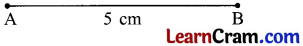(b) 7.3 cm
Solution:(c) 4.4 cm
Solution:(d) 6.7 cm
Solution: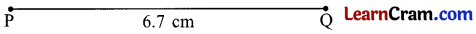Question 4.
Write two example of objects having the shape of:

(a) Cuboid
Solution:

1. Brick
2. Match stick box

(b) Circle
Solution:

1. Bangles
2. Chapati

(c) Square
Solution:

1. Photo Frame
2. Tiles.

(d) Cone
Solution:

1. Birthday cap
2. Ice cream.

(e) Cylinder
Solution:

1. Rolling pins
2. Batteries

(f) Sphere
Solution:

1. Marbles
2. BallsQuestion 5.
How many triangles are there in the given figure?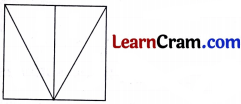Solution:
5

Question 6.
How many squares are there in the given figure?Solution:
8

Question 7.
How many line segments are there in the given figure?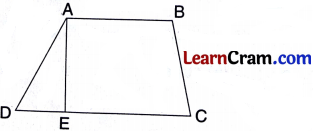Solution:
7Question 1.
Fill in the blanks.

(a) In a rectangle, the ______ sides are equal.
Solution:
opposites

(b) A circle has side and ______corners.
Solution:
no

(c) A cube has ______ faces and ____ edges.
Solution:
6, 12

(d) A sphere has 1 _______ face.
Solution:
curved

(e) A cylinder has _____ flat surface, _____ curved face ______ edges.
Solution:
2, 1, 2Question 2.
How many corners, how many sides?

(a)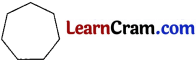Solution:
Corners – 7
sides – 7

(b)Solution:
Corners – 5
sides – 7

(c)Solution:
Corners – 4
sides – 8Question 3.
Name all the line segments in each shape.

(i)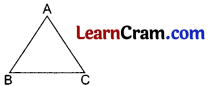Solution:
AB, AC, BC

(ii)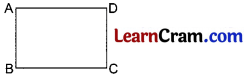Solution:

(iii)Solution:
TS, SR, RQ, PQ, TPQuestion 4.
State true or false.

(i) We can draw many lines through one point.
Solution:
True

(ii) Rolling pins and batteries are cylinder.
Solution:
True

(iii) All the edges of cuboid are equal.
Solution:
False

(iv) Sphere have one edge and one flat surface.
Solution:
False

(v) Matchbox, bricks, rooms and cupboards are not cuboids.
Solution:
FalseQuestion 5.
Draw a line segments of following length.

(a) 6 cm
Solution: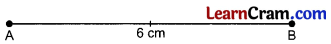(b) 2.5 cm
Solution: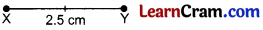(c) 4.2 cm
Solution: